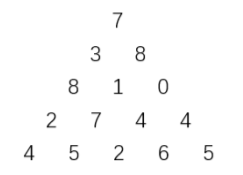【无标题】动态规划

1.动态规划题目特点：

2.解动态规划的步骤

化成子问题(最少的硬币拼出更小的面值27-Ak)

### 题目描述### 输入输出样例

`573 88 1 02 7 4 44 5 2 6 5`

`27`

`#include        #include          //注意：题目中向左下走的次数与向右下走的次数相差不能超过 1，它是最后走的次数之间的差，//并不是每走一步的差。int max(int a,int b)//求最大值{    return a>b?a:b;    }int main(int argc, char *argv[]){  int n,sum=0;  int a,f;//a数组用描述题目，f数组用来存储和值  scanf("%d",&n);  for(int i=1;i<=n;i++)    for(int j=1;j<=i;j++)      scanf("%d",&a[i][j]);//输入数据  f=a;//把开头第一个数直接给f数组  for(int i=2;i<=n;i++)//从第二行遍历开始  {      for(int j=1;j<=i;j++)      {          if(j==1) f[i][j]=f[i-1][j]+a[i][j];//每行f数组开头的数就等于a数组本身加上上一行的第一个数          else if(i==j) f[i][j]=f[i-1][j-1]+a[i][j];//末尾的数和上一行相同          else f[i][j]=max(f[i-1][j],f[i-1][j-1])+a[i][j];//重点，如果不是本身，则等于数组f的左上和右上的最大值加上数组a本身      }  }  //找规律发现如果n为奇数时，最后必然走到最后行最中间的数，如果为偶数，则取中间两个数的最大值，  //因为向左下走的次数与向右下走的次数相差不能超过 1  if(n%2==1)  printf("%d",f[n][n/2+1]);//奇数情况下  else printf("%d",max(f[n][n/2],f[n][n/2+1]));  return 0;}       `

#### 最新留言

[***.192.178.218]2023年11月17日 20时47分39秒